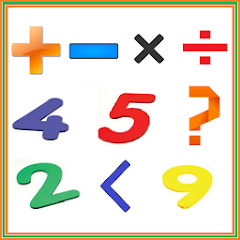# Math Game:Math Tutorial kids

50K+Everyone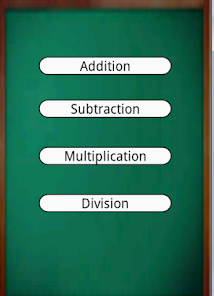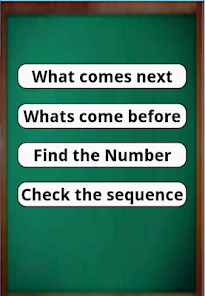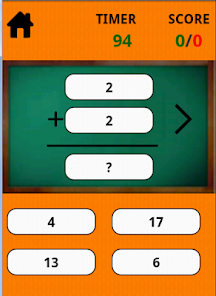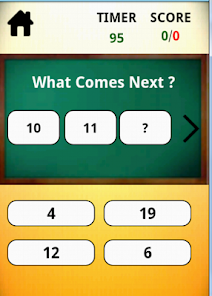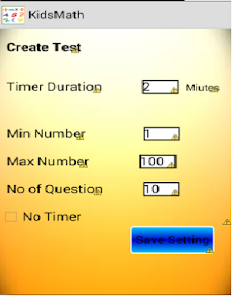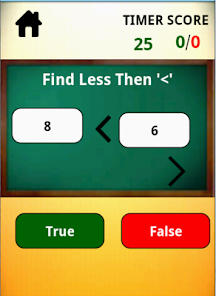Learn maths for kids easily or Kids math game for 1 grade is a free learning math tutorial by which kids learn math with fun and enjoy learning arithmetics operation like addition, subtraction, multiplication and division. This Math application also have greater then , lesser then, in which kids learn 6 is greater then 7 or lesser. Arrange the number
Another feature of this math tutorial is to find next number in sequence , guess the previous number in sequence,
guess the next number in sequence. this is something like Guessing game for kids. The whole application is like a math fun game which helps kids to revise arithmetic problems and there class work. Kids face issue in reverse counting , greate then lesser then , what comes next but this application helps kids to learn math with fun.

Kids can select different difficulty level like easy, Medium and hard and practice the math series wise and save there setting as well

Kids Math Learning point:
1. reverse counting
2. missing number
3. forward and backward counting
4. find what comes next and find what comes before
5. basic math operation
6. Less then
7. greater then
8. Arrange the number in order
9. find and arrange the lesser and greater number

IF YOU LIKE THIS APP PLEASE RATE THIS.
Updated on
Nov 5, 2016

## Data safety